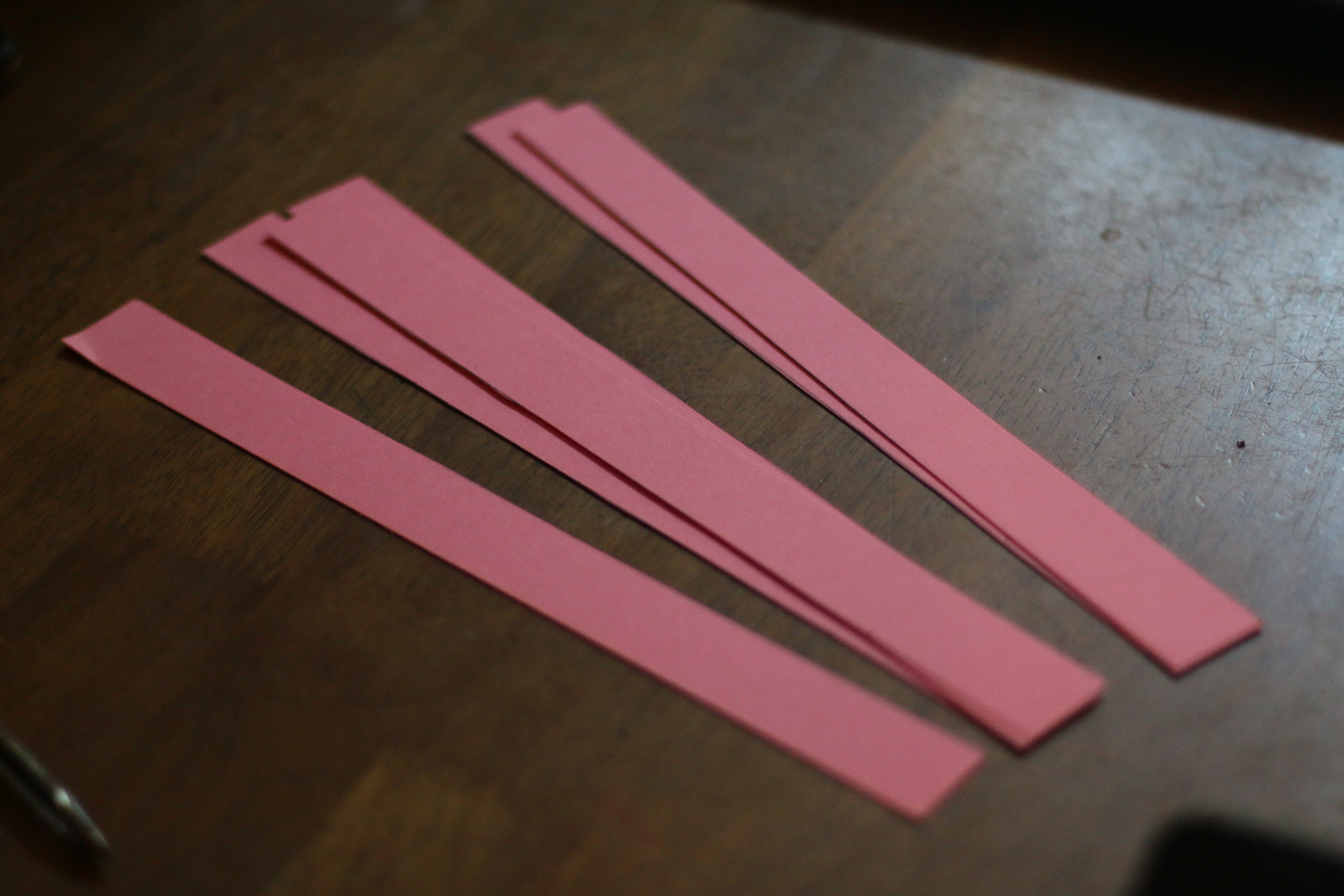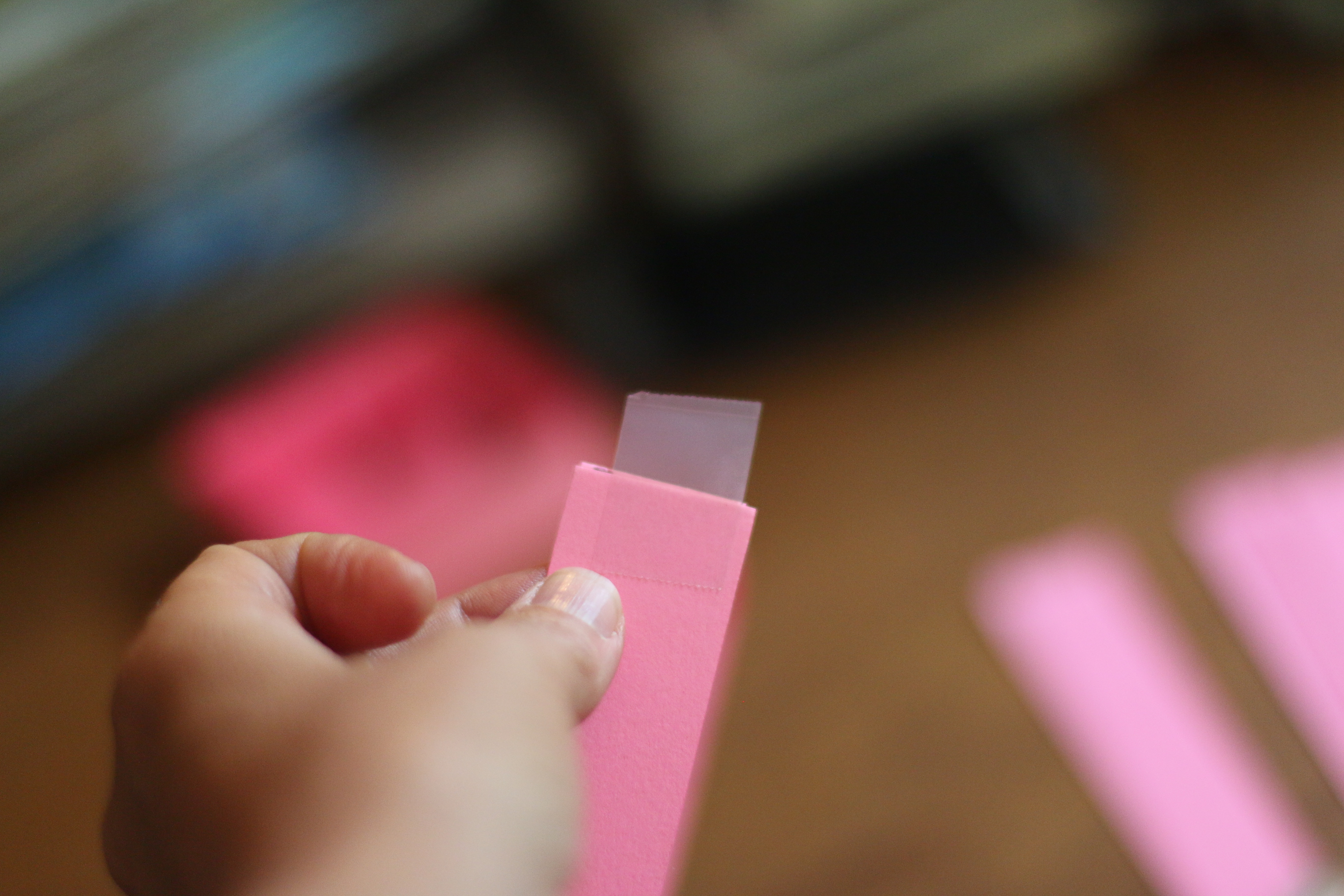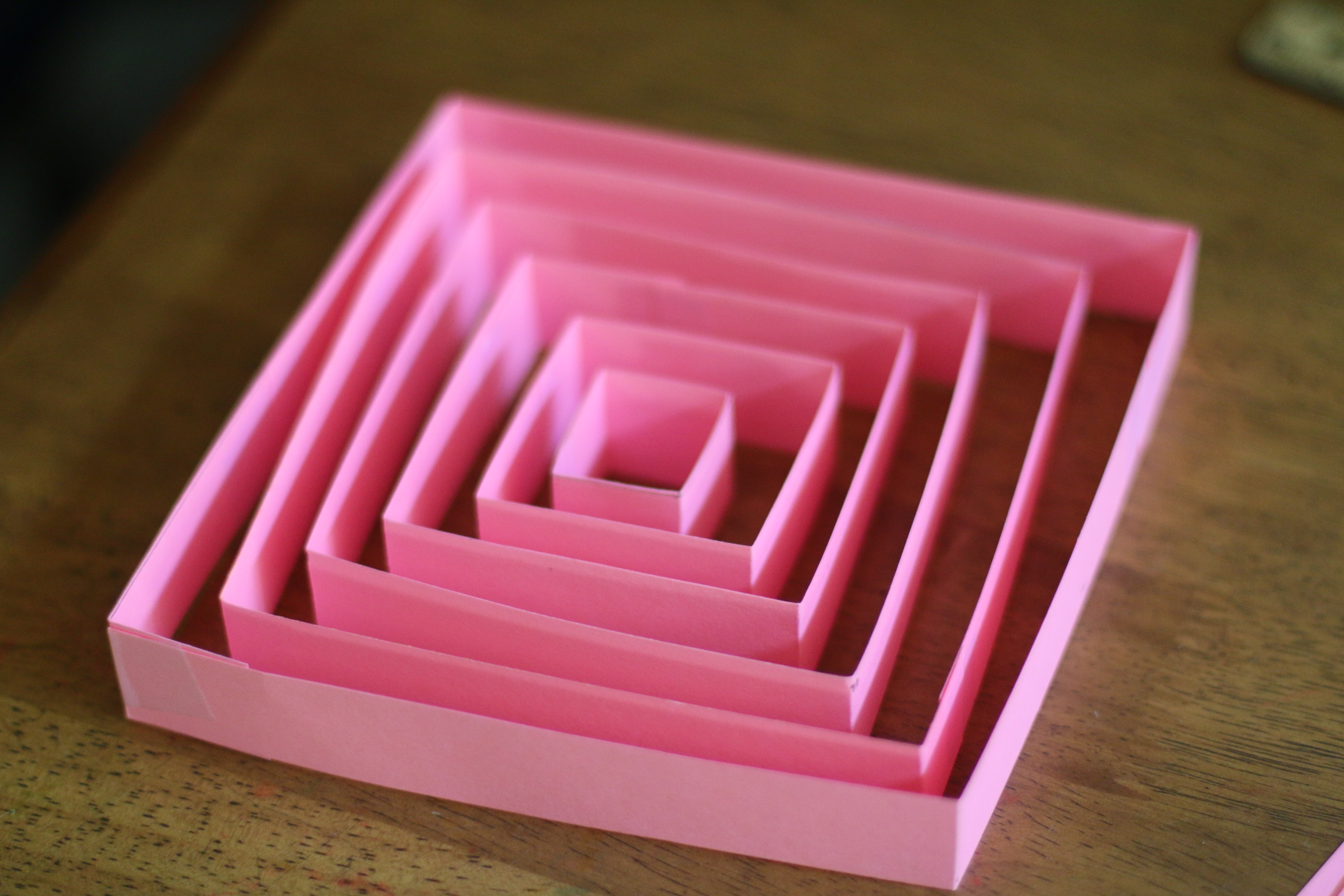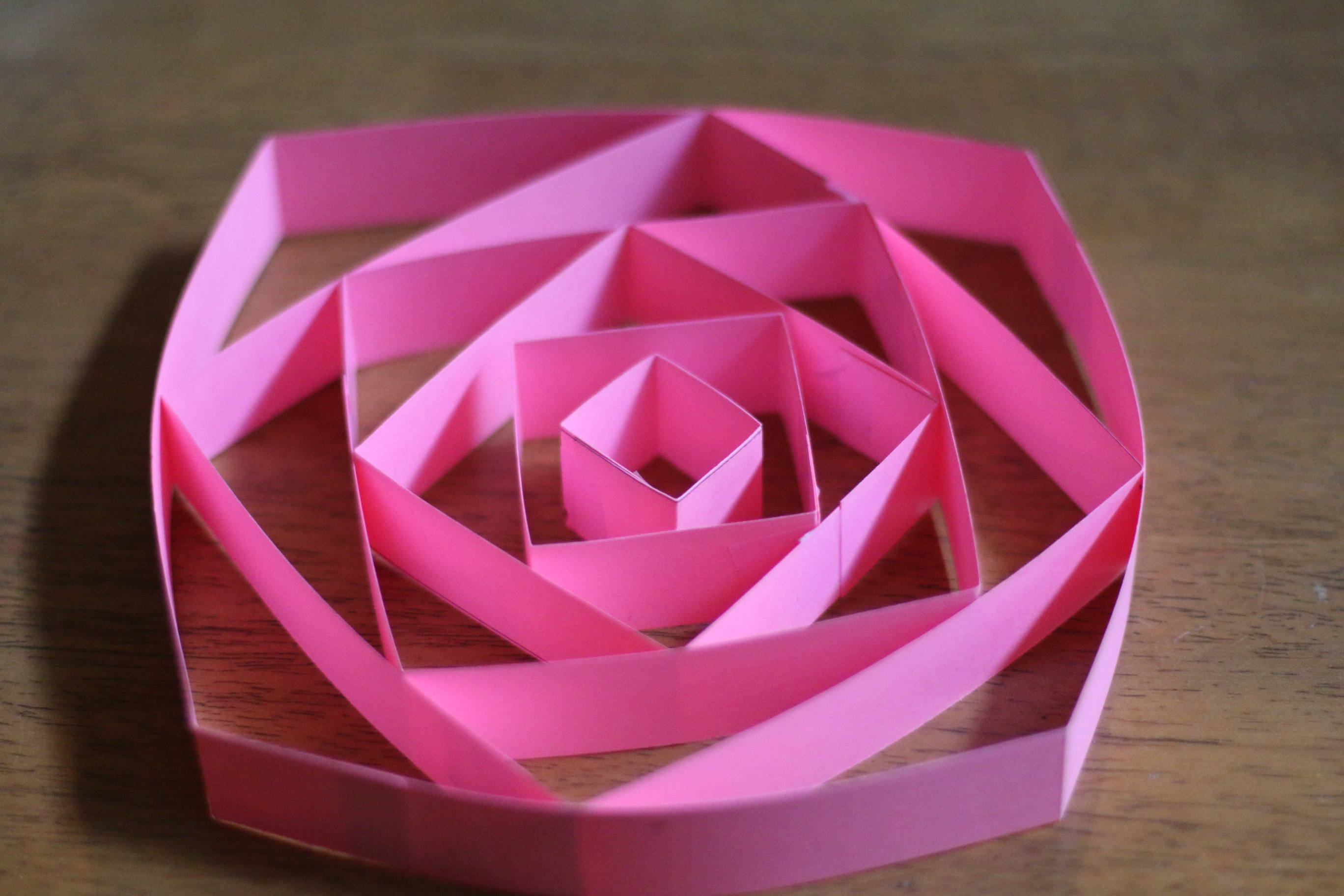# Using nesting shapes when playing with math

Nesting shapes are a fun, visual way to explore shapes and math.  In addition they lend themselves to flexibility of ages and a variety of mathematical concepts.  I’ve written a short tutorial in making them.

1. First take a piece of cardstock 8 ½ by 11 and mark 1 inch strips lengthwise. Cut out those strips.2. For squares, mark four 1 inch segments on a strip of paper.3. Cut about a ¼ inch after the last mark.
4. Score the paper at each inch mark with the scissors5. Fold along the scored marks and connect the two sides placing the ¼ inch tab on the inside and tape both inside and out to create your shape.6. Repeat steps 2 through 5 using two inch segments on the second strip, three inches on the third square, four on the fourth and so forth.

Note: On the rest of the segments, two to three strips much be taped together in order to achieve the square.  One can do this with triangles, circles, pentagons and so forth.Play in and of itself is so valuable to young children.  They learn so much just by playing, so one could just say, “How many designs do you think we can make?” and go from there.There are many concepts to be explored though.  You can look at the ratios of one shape to another.  You can look at the needed ratio for a particular design, because not all of them will fit in certain patterns.  You will notice in the  pattern where the squares fit with the vertices in the next square’s midpoints, as in the image below, they don’t fit quite right with exception to the 2 and 3 squares.  What is the ratio for these squares? What size would we need to make the next one to have a perfect fit?In the image below of the spiraling squares, the shorter piece, dissected by the smaller squares vertices,  gets smaller as it moves from the center outward. One can measure each and predict what the next will be when they understand the pattern.What are some other ideas that you have in using nesting shapes?